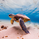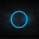‎33007‎ مشاهدة
33007
Available Options on Inputs Tab!!!

RSI with ability to change first RSI to a different Time Frame.(Defaults To Current Chart Time Frame).

Ability To Turn On/Off Background Highlighting if First RSI is Above/Below 70 or 30 Lines.

Ability To Turn On/Off Background Highlighting When First RSI Crosses Above 30 Or Below 70.

Ability To Turn On/Off "B" Or "S" When First RSI Crosses Above 30 Or Below 70.

Ability To Turn On/Off Mid -Line Plot.

Option To Plot 2nd RSI to show different Time Frames on same chart!!!

Ability To Use Different Look Back Period If You Plot 2nd RSI .
```//Created By ChrisMoody on 8/15/2014
///RSI with ability to change first RSI to a different Timeframe.
//option to Plot 2nd RSI to show different Timeframes on same chart

study(title="CM_Ultimate RSI MTF", shorttitle="CM_Ult_RSI_MTF", precision=0)
src = close
len = input(14, minval=1, title="Length")
upLine = input(70, minval=50, maxval=90, title="Upper Line Value?")
lowLine = input(30, minval=10, maxval=50, title="Lower Line Value?")
sml = input(true, title="Show Mid Line?")
sbh = input(true, title="Show Back Ground Highlights When RSI is Above/Below High/Low Lines?")
sch = input(true, title="Show Back Ground Highlights When RSI Cross?")
sl = input(true, title="Show 'B' and 'S' Letters When RSI Crosses High/Low Line?")
useCurrentRes = input(true, title="Use Current Chart Resolution?")
resCustom = input(title="Use Different Timeframe? Uncheck Box Above", type=resolution, defval="60")
ssRSI = input(false, title="Show 2nd RSI?")
resCustom2 = input(title="Use 2nd RSI? Check Box Above", type=resolution, defval="D")
useCurrentRes2 = input(false, title="Use 2nd RSI Plot On Samet Timeframe?")
len2 = input(14, minval=1, title="2nd RSI Length")

res = useCurrentRes ? period : resCustom
res2 = useCurrentRes2 ? period : resCustom2

up = rma(max(change(src), 0), len)
down = rma(-min(change(src), 0), len)
rsi = down == 0 ? 100 : up == 0 ? 0 : 100 - (100 / (1 + up / down))
outRSI = security(tickerid, res, rsi)

up2 = rma(max(change(src), 0), len2)
down2 = rma(-min(change(src), 0), len2)
rsi2 = down2 == 0 ? 100 : up2 == 0 ? 0 : 100 - (100 / (1 + up2 / down2))
outRSI2 = security(tickerid, res2, rsi2)

aboveLine = outRSI > upLine ? 1 : 0
belowLine = outRSI < lowLine ? 1 : 0
crossUp = outRSI <  lowLine and outRSI > lowLine ? 1 : 0
crossDn = outRSI >  upLine and outRSI < upLine ? 1 : 0

bgcolor(sbh and aboveLine ? red : na, transp=70)
bgcolor(sbh and belowLine ? green : na, transp=70)
bgcolor(sch and crossUp ? lime : na, transp=40)
bgcolor(sch and crossDn ? red : na, transp=40)

plot(outRSI, title="RSI", style=line, linewidth=3, color=aqua)
plot(ssRSI and outRSI2 ? outRSI2 : na, title="2nd RSI - Different Time Frame?", style=linebr, linewidth=4, color=orange)
p1 = plot(upLine, title= "Upper Line", style=solid, linewidth=3, color=red)
p2 = plot(lowLine, title= "Lower Line", style=solid, linewidth=3, color=lime)
plot(sml and 50 ? 50 : na, title="Mid Line", style=linebr, linewidth=2, color=gray)
plotchar(sl and crossUp ? crossUp : na, title="Buy Signal", char='B', location=location.bottom, color=lime, transp=0, offset=0)
plotchar(sl and crossDn ? crossDn : na, title="Sell Signal", char='S', location=location.top, color=red, transp=0, offset=0)
fill(p1, p2, color=silver, transp=70)```

## التعليقاتThis is the closest thing to the Holy Grail! Forward tested it on 2H chart for one month (June 20 - July 20 on EUR/USD - with my own settings). Buy when the RSI is crossing up the 50 line and reverse the trade at each crossing of the 50 line. And thanks Chris for all these published indicators and specially this one.
ردHaving difficulty figuring out how to set alerts with this indicator, is there a resource available on this? Thanks for the great indicator.
رد@ChrisMoody hey, one problem ive found in this indicator is, when i let the chart display the current values on the right side, the value of the Cyan coloured line is not updated correctly. Does this happen only on my chart?
رد